Updated date:

# Locally Weighted Regression(lowess)

## What is LOWESS?

In this article, we will explore one interesting algorithm which is called locally weighted regression. So you must have learned about linear regression. As can be seen in the figure below, this algorithm cannot be used to make predictions when there is a non-linear relationship between X and Y. Sometimes, it’s quadratic. Sometimes, it may be sinusoidal, and sometimes it may just be all over the place. However, if we want to predict a given point, we want our prediction to be as good as possible. How do we achieve this?

In such cases, locally weighted regression is used.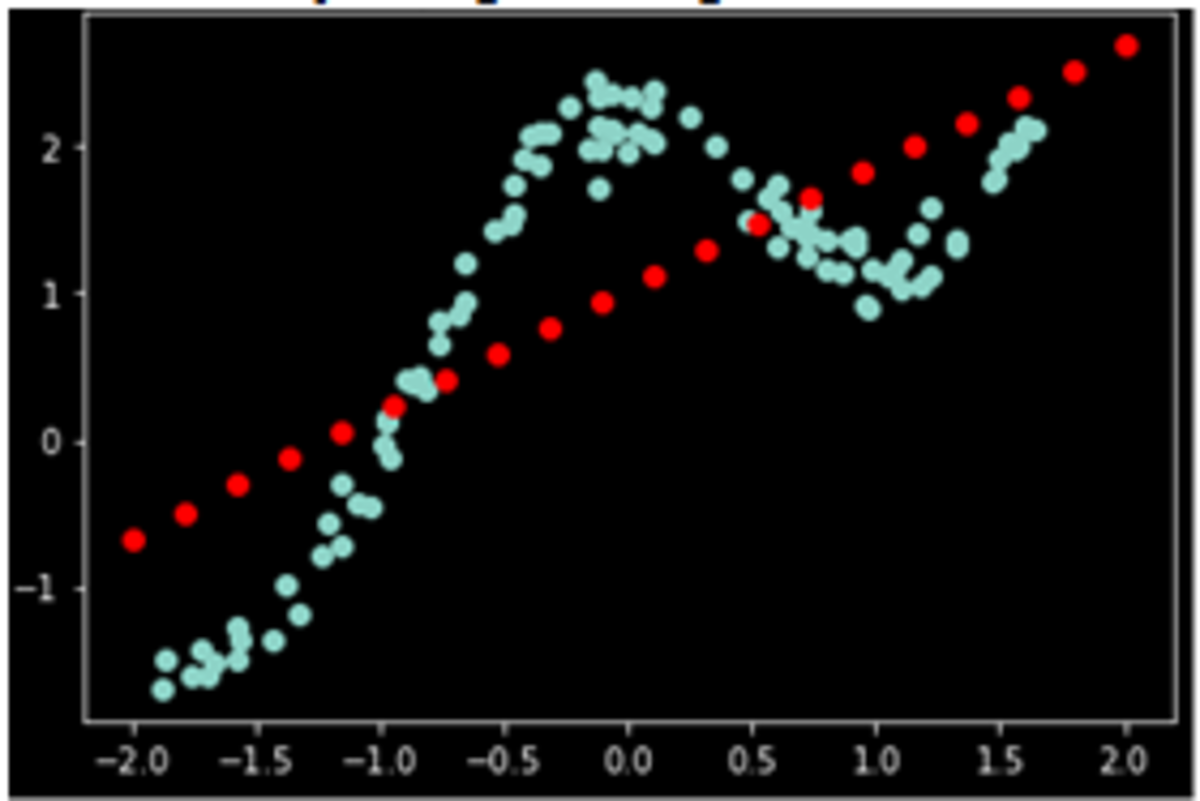So the idea of this algorithm is to give a smooth curve. This algorithm does not learn a fixed set of parameters as it is done in linear regression. Rather parameters are computed separately for each query point.

While computing parameters, a higher preference is given to the points in the training set lying in the vicinity of x than the points lying far away from the x.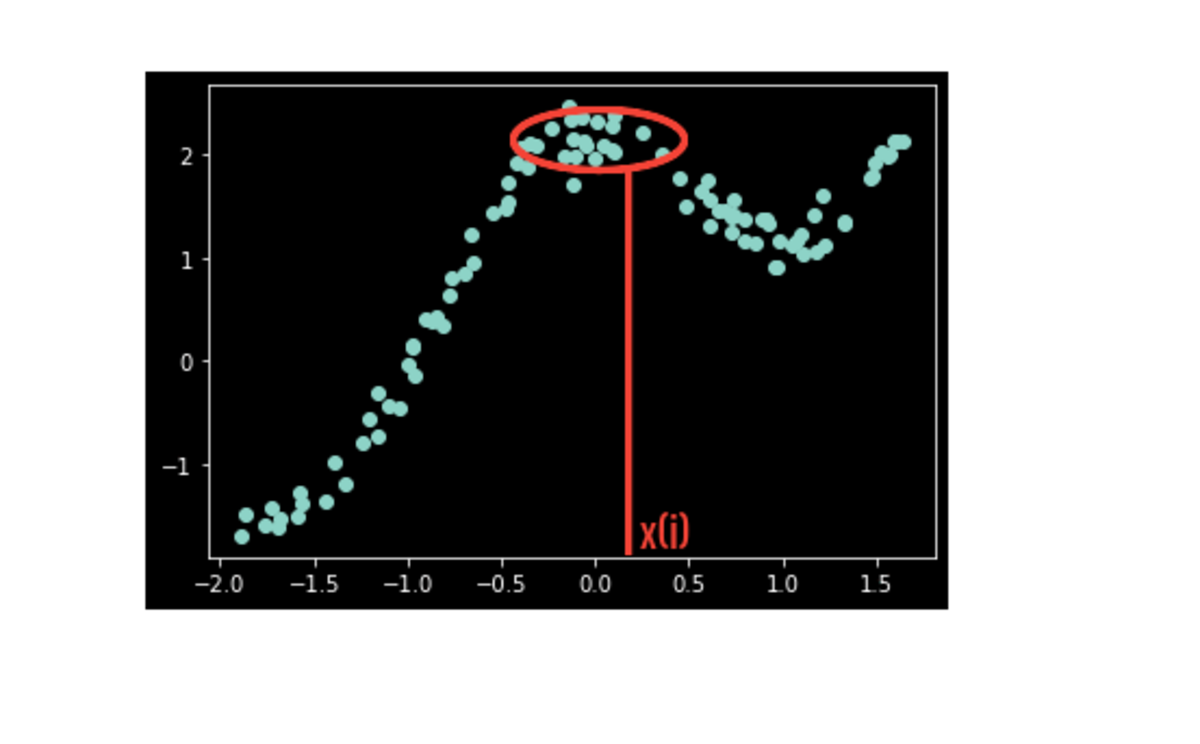The blue dots are the training data. We have a test point, x(i). Obviously, fitting one line to this whole dataset will lead to a very poor prediction. So here we will use this weighting concept and only look at a few nearby points and perform regression using those nearby points only.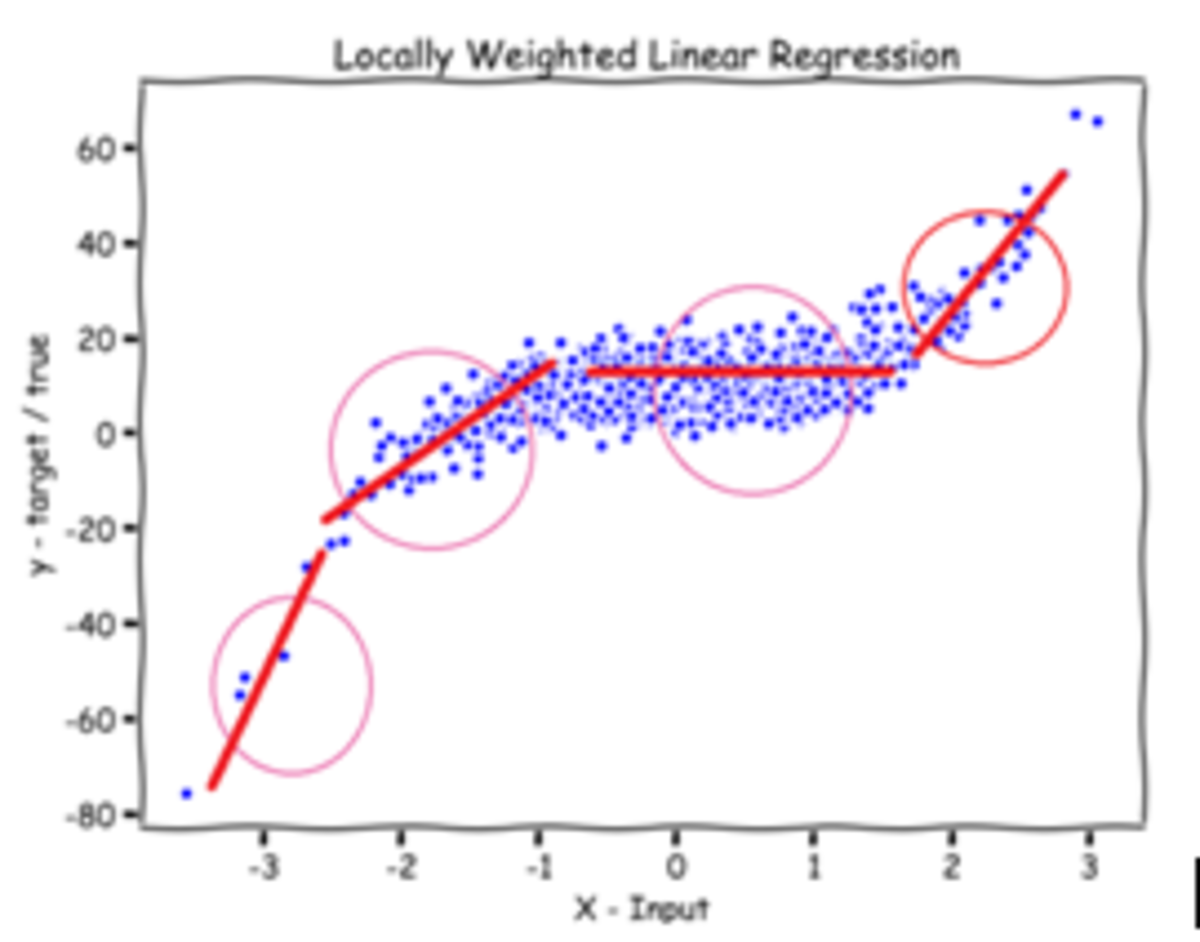So basically, we are still doing regression, but more weightage is given to the points in the training data that are close to the query point(a point we want to predict).

Here we can say, we don’t have one ready model that we can use for any new test point. For this reason, locally weighted linear regression is called a non-parametric model.

Let's now go over the math, and see how we change standard linear regression to this.

## What is Loss Function?

Loss function in the linear regression is mean squared error i.e.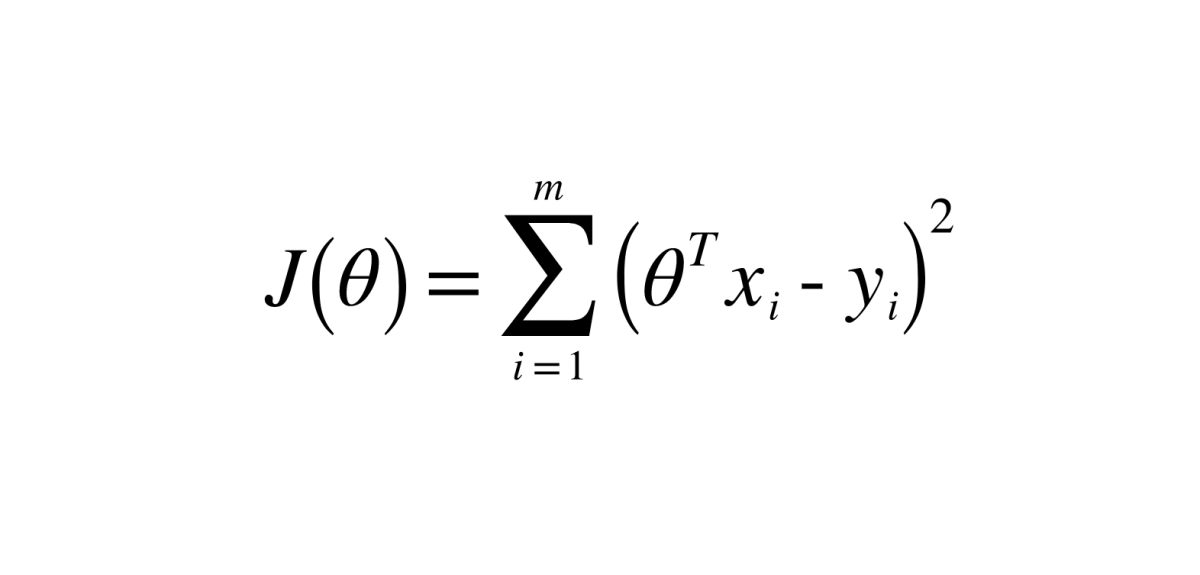The loss function, in this case, will be modified by the weighted loss function.

(here the factor of weights to each data point based on their distance from the query point will be added)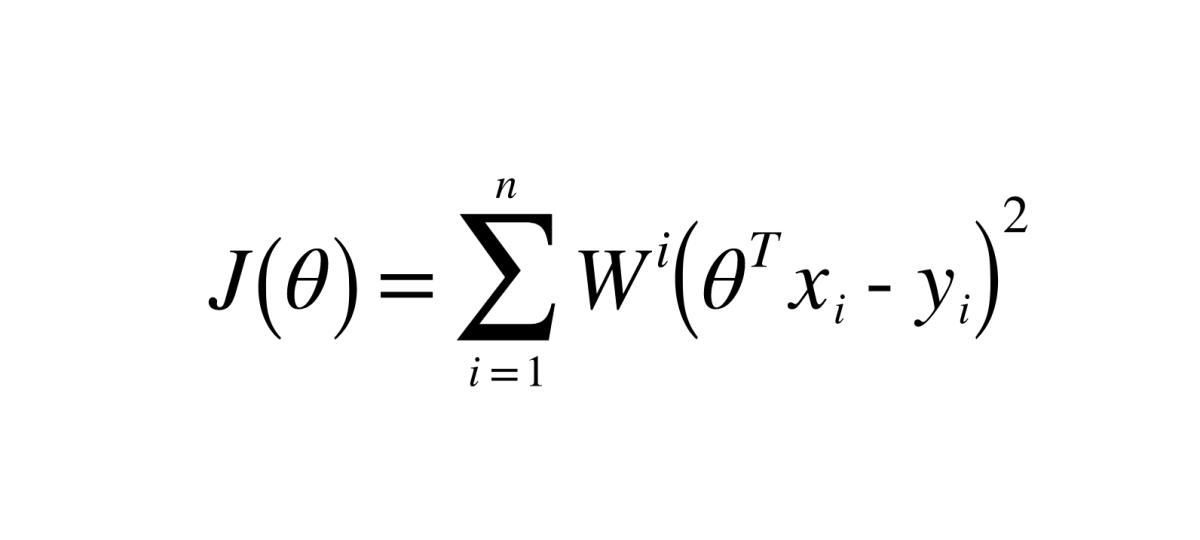Neighbors of query points will have more weights and as the distance of points increases from the query point, weights decrease.

Note- During calculations, weights must be written in the form of a diagonal matrix.

## Calculation of Weights

Weights are calculated from the exponential function which is given by –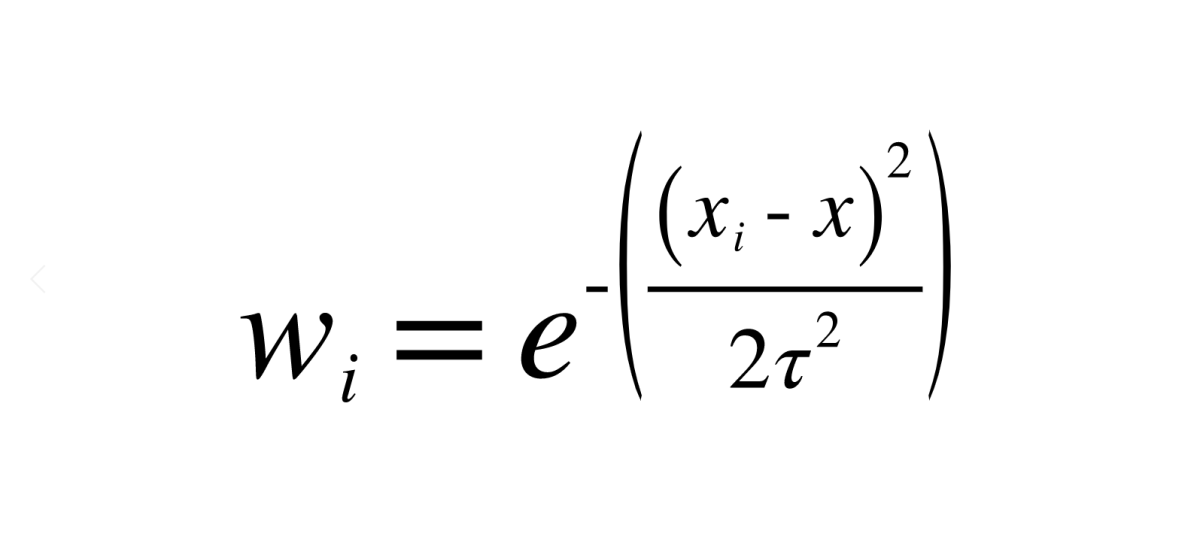X is query point

X(i) is any point in the dataset

Tau is bandwidth

Hence, from this formula, we can conclude that weights always lie between 0 and 1.

One way to look at this function is that training points near the test point, x(i)-x are small (close to 0), so the weight is close to 1. For training points far away,x(i)-x is big, so the weight term is close to 0.

## Calculating Parameters For Any Query Point

The hypothesis function of locally weighted regression remains the same as of linear regression i.e.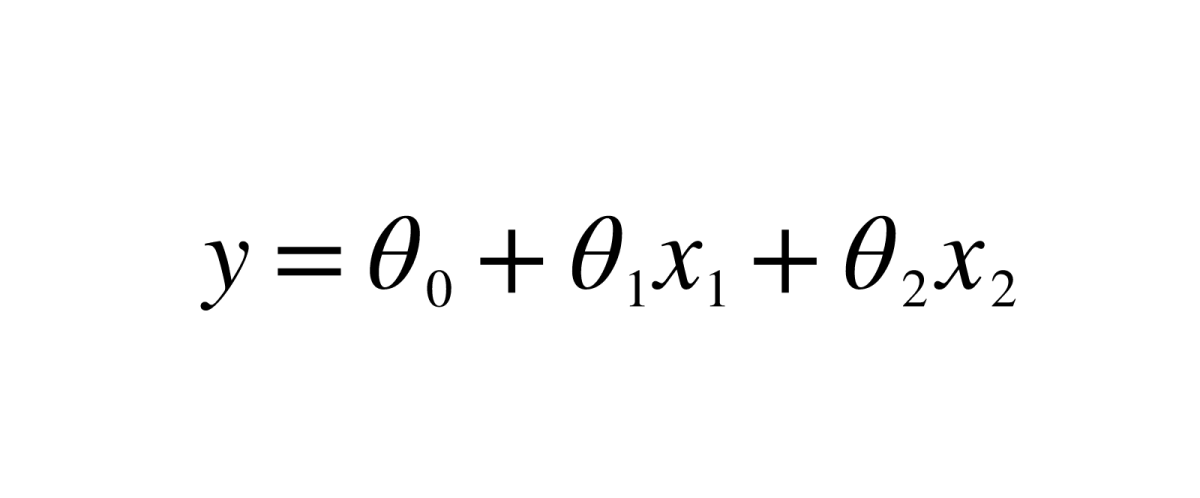Here we have to calculate the values of theta so that we can use the equation to predict values. In linear regression, it is calculated from the formula i.e.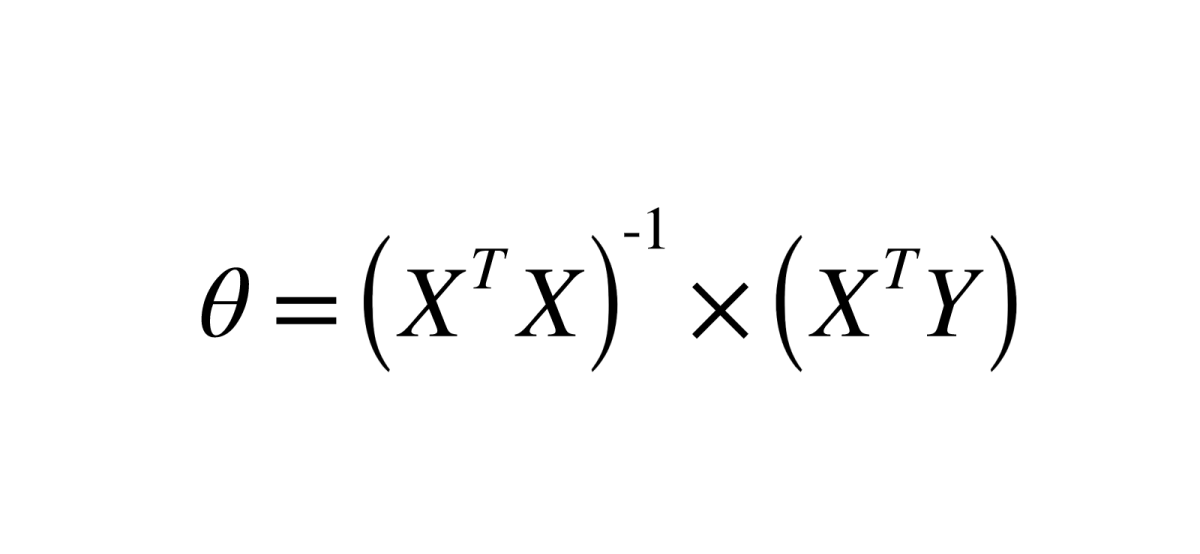## How do we get this formula?

Our motive is to minimize the loss function. So, we have to take the derivative of the loss function and equate it to zero and then find the values of theta. We will get the above-mentioned formula.

By applying the same method to locally weighted regression, the formula to calculate the values of theta we get is–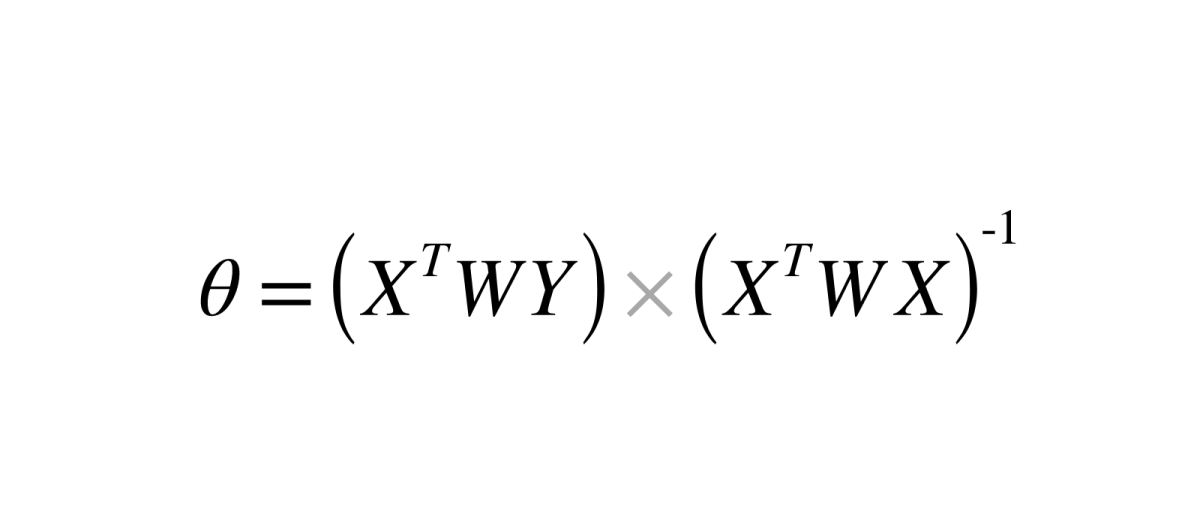Here just a factor of weights is introduced into the formula, we just saw for linear regression.

(Remember: weights are written in the form of a diagonal matrix.)

Visualization of the output of this algorithm -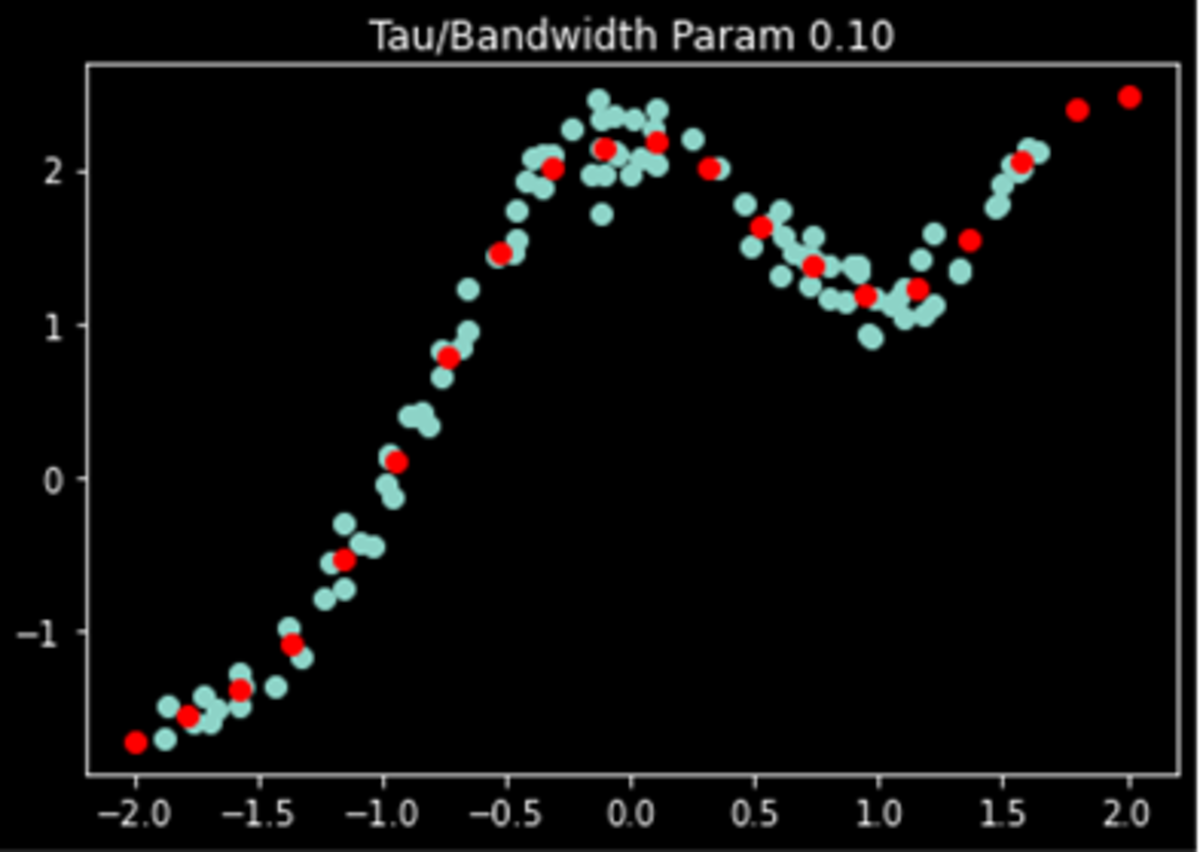## Effect of Bandwidth Parameter

As we increase the bandwidth (tau), our algorithm will converge to linear regression. Because, on increasing the value of tau, weights will tend to 1, which can be observed from the formula.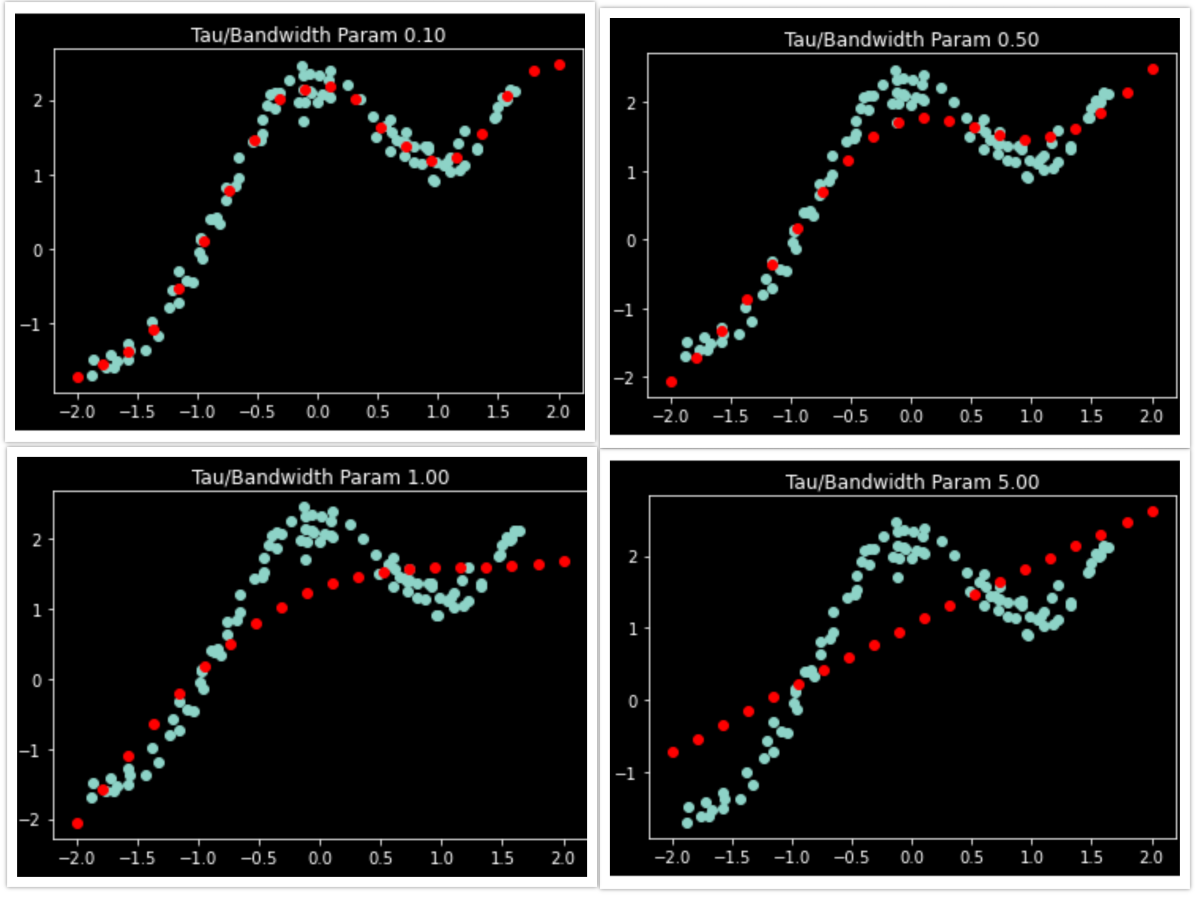## Points to remember:

• Locally weighted regression is a supervised learning algorithm.
• It is a non-parametric algorithm.
• There is no training phase. All the work is done during the testing phase/while making predictions

## Check your knowledge

For each question, choose the best answer. The answer key is below.

1. What will be the effect of bandwidth(tau) on LOWESS?
• With increase in tau, algorithm will act as linear regression.
• With decrease in tau, algorithm will act as linear regression.
2. In this algorithm, we do not have any training phase and all the work is done during the testing phase.
• TRUE
• FALSE

### Answer Key

1. With increase in tau, algorithm will act as linear regression.
2. TRUE